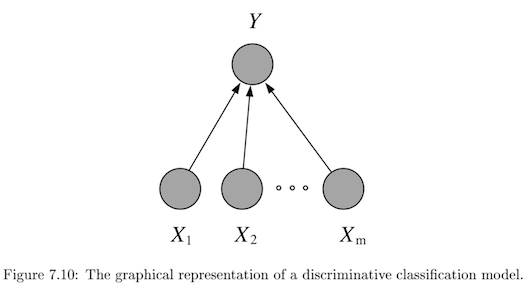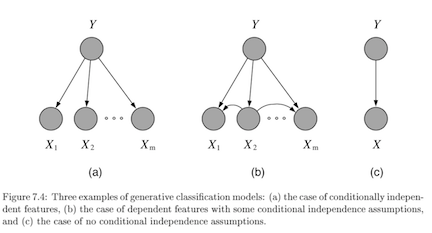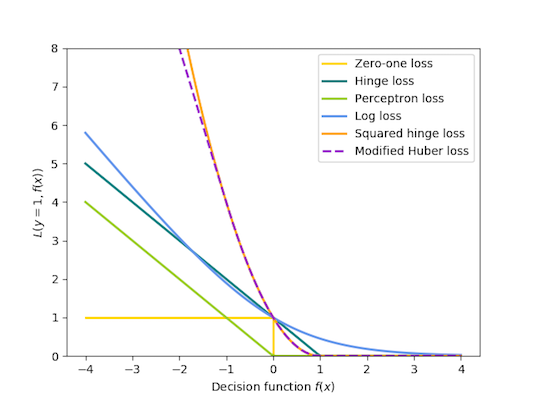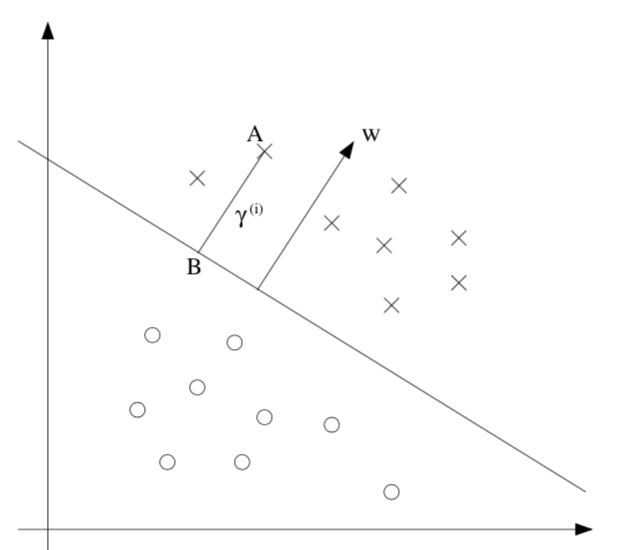# 5.5. classification¶

## 5.5.1. overview¶

• regressor doesn’t classify well

• even in binary case, outliers skew fit

• asymptotic classifier - assumes infinite data

• linear classifer $$\implies$$ boundaries are hyperplanes

• discriminative - model $$P(Y\vert X)$$ directly• usually lower bias $$\implies$$smaller asymptotic error

• slow convergence ~ $$O(p)$$

• generative - model $$P(X, Y) = P(X\vert Y) P(Y)$$• usually higher bias $$\implies$$ can handle missing data

• this is because we assume some underlying X

• fast convergence ~ $$O[\log(p)]$$

• decision theory - models don’t require finding $$p(y\|x)$$ at all

## 5.5.2. binary classification¶

• $$\hat{y} = \text{sign}(\theta^T x)$$

• usually $$\theta^Tx$$ includes b term, but generally we don’t want to regularize b

Model

$$\mathbf{\hat{\theta}}$$ objective (minimize)

Perceptron

$$\sum_i \max(0, -y_i \cdot \theta^T x_i)$$

Linear SVM

$$\theta^T\theta + C \sum_i \max(0,1-y_i \cdot \theta^T x_i)$$

Logistic regression

$$\theta^T\theta + C \sum_i \log[1+\exp(-y_i \cdot \theta^T x_i)]$$

• svm, perceptron use +1/-1, logistic use 1/0

• perceptron - tries to find separating hyperplane

• whenever misclassified, update w

• can add in delta term to maximize margin

•## 5.5.3. multiclass classification¶

• reducing multiclass (K categories) to binary

• one-against-all

• train K binary classifiers

• class i = positive otherwise negative

• take max of predictions

• one-vs-one = all-vs-all

• train $$C(K, 2)$$ binary classifiers

• labels are class i and class j

• inference - any class can get up to k-1 votes, must decide how to break ties

• flaws - learning only optimizes local correctness

• single classifier - one hot vector encoding

• multiclass perceptron (Kesler)

• if label=i, want $$\theta_i ^Tx > \theta_j^T x \quad \forall j$$

• if not, update $$\theta_i$$ and $$\theta_j$$* accordingly

• kessler construction

• $$\theta = [\theta_1 ... \theta_k]$$

• want $$\theta_i^T x > \theta_j^T x \quad \forall j$$

• rewrite $$\theta^T \phi (x,i) > \theta^T \phi (x,j) \quad \forall j$$

• here $$\phi (x, i)$$ puts x in the ith spot and zeros elsewhere

• $$\phi$$ is often used for feature representation

• define margin: $$\Delta (y,y') = \begin{cases} \delta& if \: y \neq y' \\ 0& if \: y=y'\end{cases}$$

• check if $$y=\text{argmax}_{y'} \theta^T \phi(x,y') + \delta (y,y')$$

• multiclass SVMs (Crammer & Singer)

• minimize total norm of weights s.t. true label score is at least 1 more than second best label

• multinomial logistic regression = multi-class log-linear model (softmax on outputs)

• we control the peakedness of this by dividing by stddev

## 5.5.4. discriminative¶

### 5.5.4.1. logistic regression¶

• $$p(Y=1|x, \theta) = \text{logistic}(\theta^Tx)$$

1. assume Y ~ $$\text{Bernoulli}(p)$$ with $$p=\text{logistic}(\theta^Tx$$)

2. can solve this online with GD of likelihood

3. better to solve with iteratively reweighted least squares

• $$Logit(p) = \log[p / (1-p)] = \theta^Tx$$

• multiway logistic classification

• assume $$P(Y^k=1|x, \theta) = \frac{e^{\theta_k^Tx}}{\sum_i e^{\theta_i^Tx}}$$, just as arises from class-conditional exponential family distributions

• logistic weight change represents change in odds

• fitting requires penalty on weights, otherwise they might not converge (i.e. go to infinity)

### 5.5.4.2. binary models¶

• probit (binary) regression

• $$p(Y=1|x, \theta) = \phi(\theta^Tx)$$ where $$\phi$$ is the Gaussian CDF

• pretty similar to logistic

• noise-OR (binary) model

• consider $$Y = X_1 \lor X_2 \lor … X_m$$ where each has a probability of failing

• define $$\theta$$ to be the failure probabilities

• $$p(Y=1|x, \theta) = 1-e^{-\theta^Tx}$$

• other (binary) exponential models

• $$p(Y=1|x, \theta) = 1-e^{-\theta^Tx}$$ but x doesn’t have to be binary

• complementary log-log model: $$p(Y=1|x, \theta) = 1-\text{exp}[e^{-\theta^Tx}]$$

### 5.5.4.3. decision trees / rfs - R&N 18.3; HTF 9.2.1-9.2.3¶

• importance scores

• dataset-level

• for all splits where the feature was used, measure how much variance reduced (either summed or averaged over splits)

• the sum of importances is scaled to 1

• prediction-level: go through the splits and add up the changes (one change per each split) for each features

• note: this bakes in interactions of other variables

• ours: only apply rules based on this variable (all else constant)

• why not perturbation based?

• trees group things, which can be nice

• trees are unstsable

• follow rules: predict based on prob distr. of points in same leaf you end up in

• inductive bias

• prefer small trees

• prefer tres with high IG near root

• good for certain types of problems

• instances are attribute-value pairs

• target function has discrete output values

• disjunctive descriptions may be required

• training data may have errors

• training data may have missing attributes

• greedy - use statistical test to figure out which attribute is best

• split on this attribute then repeat

• growing algorithm

1. information gain - decrease in entropy

• weight resulting branches by their probs

• biased towards attributes with many values

• use GainRatio = Gain/SplitInformation

• can incorporate SplitInformation - discourages selection of attributes with many uniformly distributed values

• sometimes SplitInformation is very low (when almost all attributes are in one category)

• might want to filter using Gain then use GainRatio

2. regression tree

• must decide when to stop splitting and start applying linear regression

• must minimize SSE

• can get stuck in local optima

• avoid overfitting

• don’t grow too deep

• early stopping doesn’t see combinations of useful attributes

• overfit then prune - proven more succesful

• reduced-error pruning - prune only if doesn’t decrease error on validation set

• $$\chi^2$$ pruning - test if each split is statistically significant with $$\chi^2$$ test

• rule post-pruning = cost-complexity pruning

1. infer the decision tree from the training set, growing the tree until the training data is fit as well as possible and allowing overfitting to occur.

2. convert the learned tree into an equivalent set of rules by creating one rule for each path from the root node to a leaf node.

• these rules are easier to work with, have no structure

3. prune (generalize) each rule by removing any preconditions that result in improving its estimated accuracy.

4. sort the pruned rules by their estimated accuracy, and consider them in this sequence when classifying subsequent instances.

• incorporating continuous-valued attributes

• choose candidate thresholds which separate examples that differ in their target classification

• just evaluate them all

• missing values

• could just fill in most common value

• also could assign values probabilistically

• differing costs

• can bias the tree to favor low-cost attributes

• ex. divide gain by the cost of the attribute

• high variance - instability - small changes in data yield changes to tree

• many trees

• bagging = bootstrap aggregation - an ensemble method

• bootstrap - resampling with replacement

• training multiple models by randomly drawing new training data

• bootstrap with replacement can keep the sampling size the same as the original size

• random forest - for each split of each tree, choose from only m of the p possible features

• smaller m decorrelates trees, reduces variance

• RF with m=p $$\implies$$ bagging

• voting

• consensus: take the majority vote

• average: take average of distribution of votes

• reduces variance, better for improving more variable (unstable) models

• adaboost - weight models based on their performance

• optimal classification trees - simultaneously optimize all splits, not one at a time

### 5.5.4.4. svms¶

• svm benefits

1. maximum margin separator generalizes well

2. kernel trick makes it very nonlinear

3. nonparametric - can retain training examples, although often get rid of many

1. at test time, can’t just store w - have to store support vectors

•• $$\hat{y} =\begin{cases} 1 &\text{if } w^Tx +b \geq 0 \\ -1 &\text{otherwise}\end{cases}$$

• $$\hat{\theta} = \text{argmin} \:\frac{1}{2} \vert \vert \theta\vert \vert ^2 \\s.t. \: y^{(i)}(\theta^Tx^{(i)}+b)\geq1, i = 1,...,m$$

• functional margin $$\gamma^{(i)} = y^{(i)} (\theta^T x +b)$$

• limit the size of $$(\theta, b)$$ so we can’t arbitrarily increase functional margin

• function margin $$\hat{\gamma}$$ is smallest functional margin in a training set

• geometric margin = functional margin / $$\vert \vert \theta \vert \vert$$

• if $$\vert \vert \theta \vert \vert =1$$, then same as functional margin

• invariant to scaling of w

• derived from maximizing margin: $$$\max \: \gamma \\\: s.t. \: y^{(i)} (\theta^T x^{(i)} + b) \geq \gamma, i=1,..,m\\ \vert \vert \theta\vert \vert =1$$$

• difficult to solve, especially because of $$\vert \vert w\vert \vert =1$$ constraint

• assume $$\hat{\gamma}=1$$ ~ just a scaling factor

• now we are maximizing $$1/\vert \vert w\vert \vert$$

• soft margin classifier - lets examples fall on wrong side of decision boundary

• assigns them penalty proportional to distance required to move them back to correct side

• min $$\frac{1}{2}||\theta||^2 \textcolor{blue}{ + C \sum_i^n \epsilon_i} \\s.t. y^{(i)} (\theta^T x^{(i)} + b) \geq 1 \textcolor{blue}{- \epsilon_i}, i=1:m \\ \textcolor{blue}{\epsilon_i \geq0, 1:m}$$

• large C can lead to overfitting

• benefits

• number of parameters remains the same (and most are set to 0)

• we only care about support vectors

• maximizing margin is like regularization: reduces overfitting

• actually solve dual formulation (which only requires calculating dot product) - QP

• replace dot product $$x_j \cdot x_k$$ with kernel function $$K(x_j, x_k)$$, that computes dot product in expanded feature space

• linear $$K(x,z) = x^Tz$$

• polynomial $$K (x, z) = (1+x^Tz)^d$$

• radial basis kernel $$K (x, z) = \exp(-r\vert \vert x-z\vert \vert ^2)$$

• transforming then computing is O($$m^2$$), but this is just $$O(m)$$

• practical guide

• use m numbers to represent categorical features

• scale before applying

• fill in missing values

• start with RBF

• valid kernel: kernel matrix is Psd

### 5.5.4.5. decision rules¶

• if-thens, rule can contain ands

• good rules have large support and high accuracy (they tradeoff)

• decision list is ordered, decision set is not (requires way to resolve rules)

• most common rules: Gini - classification, variance - regression

• ways to learn rules

• oneR - learn from a single feature

• sequential covering - iteratively learn rules and then remove data points which are covered

• ex rule could be learn decision tree and only take purest node

• bayesian rule lists - use pre-mined frequent patterns

• generally more interpretable than trees, but doesn’t work well for regression

• features often have to be categorical

• rulefit

• learns a sparse linear model with the original features and also a number of new features that are decision rules

• train trees and extract rules from them - these become features in a sparse linear model

• feature importance becomes a little stickier….

## 5.5.5. generative¶

### 5.5.5.1. gaussian class-conditioned classifiers¶

• binary case: posterior probability $$p(Y=1|x, \theta)$$ is a sigmoid $$\frac{1}{1+e^{-z}}$$ where $$z = \beta^Tx+\gamma$$

1. multiclass extends to softmax function: $$\frac{e^{\beta_k^Tx}}{\sum_i e^{\beta_i^Tx}}$$ - $$\beta$$s can be used for dim reduction

• probabilistic interpretation

• assumes classes are distributed as different Gaussians

• it turns out this yields posterior probability in the form of sigmoids / softmax

• only a linear classifier when covariance matrices are the same (LDA)

1. otherwise a quadratic classifier (like QDA) - decision boundary is quadratic

2. MLE for estimates are pretty intuitive

3. decision boundary are points satisfying $$P(C_i\vert X) = P(C_j\vert X)$$

• regularized discriminant analysis - shrink the separate covariance matrices towards a common matrix

• $$\Sigma_k = \alpha \Sigma_k + (1-\alpha) \Sigma$$

• parameter estimation: treat each feature attribute and class label as random variables

• assume distributions for these

• for 1D Gaussian, just set mean and var to sample mean and sample var

• can use directions for dimensionality reduction (class-separation)

### 5.5.5.2. naive bayes classifier¶

• assume multinomial Y

• with clever tricks, can produce $$P(Y^i=1|x, \eta)$$ again as a softmax

• let $$y_1,...y_l$$ be the classes of Y

• want Posterior $$P(Y\vert X) = \frac{P(X\vert Y)(P(Y)}{P(X)}$$

• MAP rule - maximum a posterior rule

• use prior P(Y)

• given x, predict $$\hat{y}=\text{argmax}_y P(y\vert X_1,...,X_p)=\text{argmax}_y P(X_1,...,X_p\vert y) P(y)$$

• generally ignore constant denominator

• naive assumption - assume that all input attributes are conditionally independent given y

• $$P(X_1,...,X_p\vert Y) = P(X_1\vert Y)\cdot...\cdot P(X_p\vert Y) = \prod_i P(X_i\vert Y)$$

• learning

• learn L distributions $$P(y_1),P(y_2),...,P(y_l)$$

• for i in 1:$$\vert Y \vert$$

• learn $$P(X \vert y_i)$$

• for discrete case we store $$P(X_j\vert y_i)$$, otherwise we assume a prob. distr. form

• naive: $$\vert Y\vert \cdot (\vert X_1\vert + \vert X_2\vert + ... + \vert X_p\vert )$$ distributions

• otherwise: $$\vert Y\vert \cdot (\vert X_1\vert \cdot \vert X_2\vert \cdot ... \cdot \vert X_p\vert )$$

• smoothing - used to fill in 0s

• $$P(x_i\vert y_j) = \frac{N(x_i, y_j) +1}{N(y_j)+\vert X_i\vert }$$

• then, $$\sum_i P(x_i\vert y_j) = 1$$

### 5.5.5.3. exponential family class-conditioned classifiers¶

1. includes Gaussian, binomial, Poisson, gamma, Dirichlet

2. $$p(x|\eta) = \text{exp}[\eta^Tx - a(\eta)] h(x)$$

3. for classification, anything from exponential family will result in posterior probability that is logistic function of a linear function of x

### 5.5.5.4. text classification¶

• bag of words - represent text as a vector of word frequencies X

• remove stopwords, stemming, collapsing multiple - NLTK package in python

• assumes word order isn’t important

• can store n-grams

• multivariate Bernoulli: $$P(X\vert Y)=P(w_1=true,w_2=false,...\vert Y)$$

• multivariate Binomial: $$P(X\vert Y)=P(w_1=n_1,w_2=n_2,...\vert Y)$$

• this is inherently naive

• time complexity

• training O(n*average_doc_length_train+$$\vert c\vert \vert dict\vert$$)

• testing O($$\vert Y\vert$$ average_doc_length_test)

• implementation

• have symbol for unknown words

• underflow prevention - take logs of all probabilities so we don’t get 0

• $$y = \text{argmax }log \:P(y) + \sum_i log \: P(X_i\vert y)$$

## 5.5.6. instance-based (nearest neighbors) - really discriminative¶

• also called lazy learners = nonparametric models

• make Voronoi diagrams

• can take majority vote of neighbors or weight them by distance

• distance can be Euclidean, cosine, or other

• should scale attributes so large-valued features don’t dominate

• Mahalanobois distance metric accounts for covariance between neighbors

• in higher dimensions, distances tend to be much farther, worse extrapolation

• sometimes need to use invariant metrics

• ex. rotate digits to find the most similar angle before computing pixel difference

• could just augment data, but can be infeasible

• computationally costly so we can approximate the curve these rotations make in pixel space with the invariant tangent line

• stores this line for each point and then find distance as the distance between these lines

• finding NN with k-d (k-dimensional) tree

• balanced binary tree over data with arbitrary dimensions

• each level splits in one dimension

• might have to search both branches of tree if close to split

• finding NN with locality-sensitive hashing

• approximate

• make multiple hash tables

• each uses random subset of bit-string dimensions to project onto a line

• union candidate points from all hash tables and actually check their distances

• comparisons

• error rate of 1 NN is never more than twice that of Bayes error

## 5.5.7. likelihood calcs¶

### 5.5.7.1. single Bernoulli¶

• $$L(p) = P$$[Train | Bernoulli(p)]= $$P(X_1,...,X_n\vert p)=\prod_i P(X_i\vert p)=\prod_i p^{X_i} (1-p)^{1-X_i}$$

• $$=p^x (1-p)^{n-x}$$ where x = $$\sum x_i$$

• $$\log[L(p)] = \log[p^x (1-p)^{n-x}]=x \log(p) + (n-x) \log(1-p)$$

• $$0=\frac{dL(p)}{dp} = \frac{x}{p} - \frac{n-x}{1-p} = \frac{x-xp - np+xp}{p(1-p)}=x-np$$

• $$\implies \hat{p} = \frac{x}{n}$$

### 5.5.7.2. multinomial¶

• $$L(\theta) = P(x_1,...,x_n\vert \theta_1,...,\theta_p) = \prod_i^n P(d_i\vert \theta_1,...\theta_p)=\prod_i^n factorials \cdot \theta_1^{x_1},...,\theta_p^{x_p}$$- ignore factorials because they are always same

• require $$\sum \theta_i = 1$$

• $$\implies \theta_i = \frac{\sum_{j=1}^n x_{ij}}{N}$$ where N is total number of words in all docs

## 5.5.8. boosting¶

1. adaboost

1. freund & schapire

2. reweight data points based on errs of previous weak learners, then train new classifiers

3. classify as an ensemble

2. gradient boosting

1. leo breiman

2. actually fits the residual errors made by the previous predictors

3. newer algorithms for gradient boosting (speed / approximations)

1. xgboost (2014 - popularized around 2016)

1. implementation of gradient boosted decision trees designed for speed and performance

2. things like caching, etc.

2. light gbm (2017)

1. can get gradient of each point wrt to loss - this is like importance for point (like weights in adaboost)

1. when picking split, filter out unimportant points

3. Catboost (2017)

4. boosting with different cost function ($$y \in \{-1, 1\}$$, or for $$L_2$$Boost, also $$y \in \mathbb R$$)

Adaboost

LogitBoost

$$L_2$$Boost

$$\exp(y\hat{y})$$

$$\log_2 (1 + \exp(-2y \hat y ))$$

$$(y - \hat y)^2 / 2$$